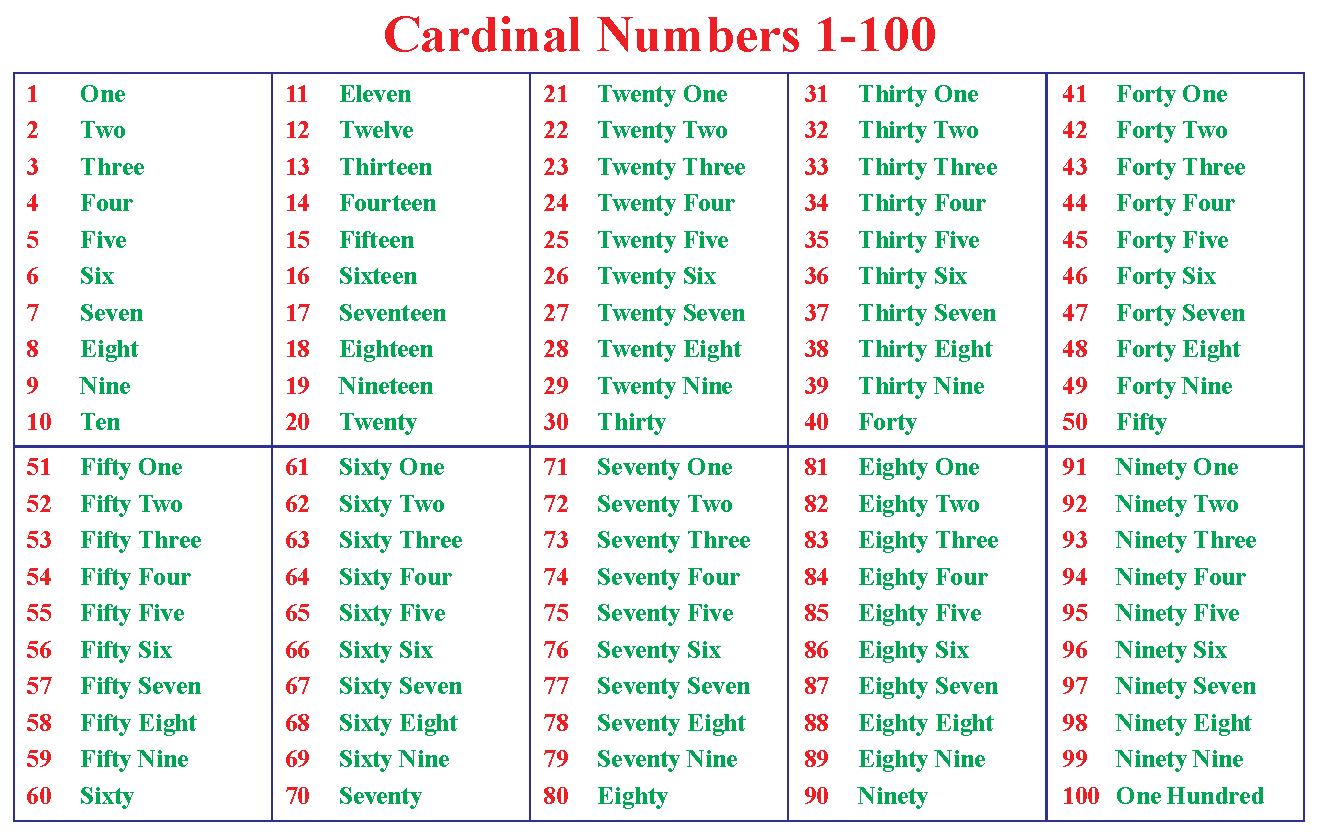# Cardinal Numbers

Cardinal Numbers
Go back to  'Numbers'

In this mini-lesson, we will explore the world of cardinal numbers and understand the difference between cardinal and ordinal numbers.

Tap on the arrow keys in the simulation below.

What do you see?

A panda climbing up / down on the steps?

The number of steps the panda climbs is represented by the cardinal number.

The position of the panda on the step (1st, 2nd, 3rd, 4th, 5th.. so on) is represented by the ordinal number.

Do you also want to know more about ordinal and cardinal numbers?

## What are Cardinal Numbers?

A Cardinal Number describes or represents how many of something are present. Example two apples, 5 flowers, etc. It quantifies an object.

It does not have values as fractions or decimals.

Cardinal numbers are counting numbers, they help to count the number of items.

Let's have a look at cardinal numbers examples.

Ana wants to count the number of people standing in a queue at a billing counter. Can you help her?

Ana started to count using Natural numbers.Ana counted 1, 2, 3, 4, and 5

There are 5 people standing in a queue at the billing counter.

Counting numbers are cardinal numbers!

Now, Let's consider another example,

Noah kept eight apples in a basket.The number eight denotes how many apples are there in the basket, irrespective of their order.

## What Is the Difference Between Cardinal and Ordinal Numbers?

Any natural number such as 1, 2, 3, etc, is referred as a cardinal number.

Cardinal numbers are used for counting.

An ordinal number is a number that denotes the position or place of an object.

Example: 1st, 2nd, 3rd, 4th, 5th, etc.

Ordinal numbers are used for ranking.

Here is an example which explains Cardinal and Ordinal Numbers:In the above image, we can see a team of 5 workers on the construction site.

This is an example of cardinal numbers.In the above image, we can see the position of the runners in the running event.

First, second, third, and so on.

This is an example of ordinal numbers.

We'll discuss more of ordinal and cardinal numbers examples in the sections below.Think Tank
1. Maria wrote January 1, 2020, as today’s date in her notebook. Does the number 1 in the date, represent a cardinal number?
2. There are _______ people in the line ahead of me. I am the 7th person in the line.

## List of Cardinal Numbers from 1 to 100

Given below are the basic and most important cardinal numbers, which form the base for other counting numbers.

 1 - One 6 - Six 2 - Two 7 - Seven 3 - Three 8 - Eight 4 - Four 9 - Nine 5 - Five 10 - Ten

 10 - Ten 60 - Sixty 20 - Twenty 70 - Seventy 30 - Thirty 80 - Eighty 40 - Forty 90 - Ninety 50 - Fifty 100 - HundredImportant Notes
• Cardinal numbers help us to count the number of things or people in or around a place or a group.
• The collection of all the ordinal numbers can be denoted by the cardinal.
• Cardinal numbers can be written as words such as one, two, three etc.
• Cardinal numbers tell how many items, whereas ordinal numbers show position or ranking.

## Solved Examples

 Example 1

Kate has a list of numbers as shown.Identify the cardinal numbers.

Solution

7, 10 and two help us in counting, whereas 8th, fourth and 2nd helps us in identifying the position.

Thus, 7, 10, and two are cardinal numbers.

 $$\therefore$$ 7, 10 and two are cardinal numbers.
 Example 2

Help Ryan, to calculate the number of vowels in "NUMBERS"

Also, identify the number of alphabets used to form this word.

Solution

(i) We know that a, e, i, o, and u are the vowels and in the given word u and e are used.

$$\therefore$$ 2 vowels are used to form this number

(ii)  We start counting from N to S, we see that "Numbers" has 7 alphabets in all.

 $$\therefore$$ Vowels = 2, total alphabets = 7
 Example 3

Look at the picture below.How many parking slots are occupied? Is your answer a cardinal number or ordinal number?

Solution

6 parking slots are occupied.

6 is a cardinal number.

 $$\therefore$$ 6 parking slots are occupied. 6 is a cardinal number.

## Interactive Questions

Here are a few activities for you to practice. Select/Type your answer and click the "Check Answer" button to see the result.

## Let's Summarize

The mini-lesson targeted in the fascinating concept of cardinal numbers. The math journey around cardinal numbers starts with what a student already knows, and goes on to creatively crafting a fresh concept in the young minds. Done in a way that not only it is relatable and easy to grasp, but also will stay with them forever. Here lies the magic with Cuemath.

At Cuemath, our team of math experts is dedicated to making learning fun for our favorite readers, the students!

Through an interactive and engaging learning-teaching-learning approach, the teachers explore all angles of a topic.

Be it worksheets, online classes, doubt sessions, or any other form of relation, it’s the logical thinking and smart learning approach that we at Cuemath believe in.

## 1. Is zero a cardinal number?

Zero is not a cardinal number. Since zero means nothing, it represents or describes no quantity or set.

## 2. Is 19 a cardinal number?

Yes, 19 is a cardinal number.

## 3. What is the biggest cardinal number?

There are infinite natural numbers. Therefore, there are as many cardinal numbers as natural numbers. There can be no generalization of the biggest natural number and so does for biggest cardinal number.

More Important Topics
Numbers
Algebra
Geometry
Measurement
Money
Data
Trigonometry
Calculus